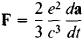(redirected from Self-force)
The following article is from The Great Soviet Encyclopedia (1979). It might be outdated or ideologically biased.

the force that acts on an electron or other charged particle owing to the electromagnetic radiation field induced by the particle.

Any motion of a charge with acceleration results in the emission of electromagnetic waves. A system of charges moving with acceleration is therefore not closed: energy and momentum are not conserved in it. Such a system is a dissipative system and behaves like a mechanical system in which frictional forces are present. The frictional forces are introduced to describe the nonconservation of energy in the system because of the system’s interaction with its surroundings. In precisely the same way, the transfer of energy and momentum by the charged particle to the electromagnetic radiation field may be described as “radiation damping.” If we know the energy lost per unit time—that is, the radiation intensity (seeRADIATION, ELECTROMAGNETIC)—we can determine the frictional force. For an electron moving in a bounded region of space with a mean speed that is small compared to the speed of light c, the frictional force is expressed by a formula first derived by H. Lorentz:where a is the acceleration of the electron. The radiation reaction leads to the damping of charge oscillations; the damping is evidenced in the broadening of the spectral line of the radiation. What is called the natural line width is the width of the line after all causes of broadening other than radiation damping have been removed.

The radiation reaction is a part of the force acting on a charge owing to the electromagnetic field created by the charge. The necessity of allowing for this reaction raises fundamental difficulties that are closely associated with such questions as the structure of the electron and the nature of the electron’s mass (see QUANTUM FIELD THEORY).

A rigorous formulation of the problem requires that the dynamic system of charges and an electromagnetic field be considered. Such a system is described by two systems of equations—the equations of the motion of the particles in the field and the equations of the field defined by the position and motion of the charged particles. In practice, however, only an approximate formulation of the problem by the method of successive approximations is feasible. For example, first the motion of an electron in the given field is found—that is, without allowance for the radiation field. Then the charge’s radiation field is found from the charge’s given motion. Finally, as a correction, the effect of this field on the motion of the charge—that is, the radiation reaction—is determined. This method yields good results for radiation of wavelength λ ≫ r0 = e2/mc2, where m is the mass of the electron and r0 ≈ 2 × 10–13 cm is the classical electron radius. Quantum effects must be taken into account for a wavelength of the order of the Compton wavelength of the electron h/mc, where h is Planck’s constant—that is, for a wavelength λ ~ 10–10 cm. The approximate method of allowing for the radiation reaction is therefore valid throughout the range of applicability of classical electrodynamics.

In a fundamental sense quantum electrodynamics has retained the same approach to the problem: the perturbation theory methods it uses involve successive approximations. Its techniques, however, permit the radiation reaction—that is, the action of the radiation field on the electron—to be taken into account with practically any degree of accuracy. This applies not only to the “dissipative” part of the radiation reaction (which is responsible for the broadening of spectral lines) but also to the “potential” part—that is, the effective change in the external field in which the electron is moving. This damping is manifested in changes in energy levels and the effective cross sections of collision processes (see).

REFERENCES

Landau, L. D., and E. M. Lifshits. Teoriia polia, 4th ed. Moscow, 1962. (Teorelicheskaia fizika, vol. 2.)
Becker, R. Elektronnaia teoriia. Leningrad-Moscow, 1936. (Translated from German.)

V. B. BERESTETSKII